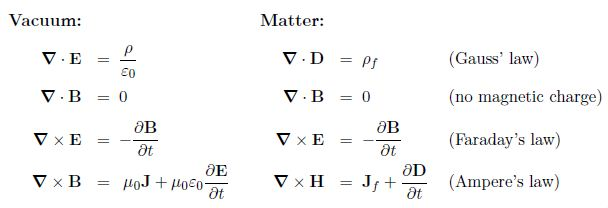Mathematics

# Maxwell’s Equations in Vacuum

Maxwell modified Ampere’s law in 1865 to make it apply to electrodynamics by adding the displacement current to the current. Replacing Ɛ E with D and B/μ0 with H we obtain versions applicable to matter. The sets of four equations of electrodynamics thus modified are known as Maxwell’s equations:These four equations together with the Lorentz force law F = q(E+ v x B) encapsulate classical electrodynamics.